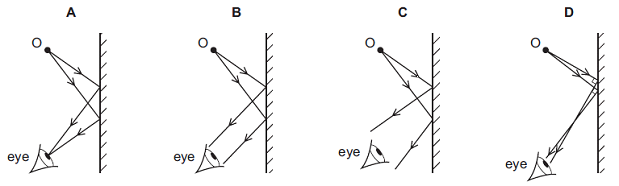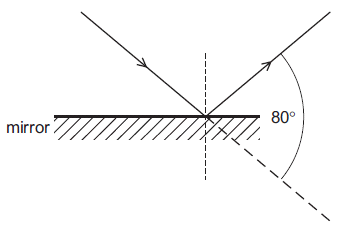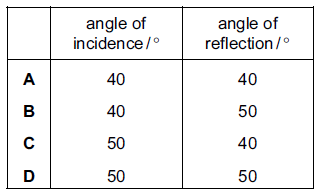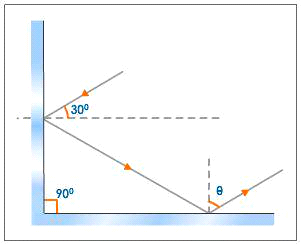PHYSICS FORM1

ONLINE TEST SERIES

RECTILINEAR PROPAGATION OF LIGHT

1. An eye views an object O by reflection in a plane mirror. Which is the correct ray diagram?2. Which of the following is used to make a periscope?

3. An object is placed 1.5 m from a plane mirror. How far is the image from the person?

4. An object placed 2m from a plane mirror is shifted by 0.5 m away from the mirror. What is the distance between the object and its image?

5. What is the angle between the incident and reflected rays when a ray of light is incident normally on a plane mirror?

6. Light is incident on a mirror and is reflected as shown.What is the angle of incidence and the angle of reflection?7. Light is a form of energy produced by a ______.

8. What is the value of è in the following diagram?9. An example for non-luminous object is ___________.

10. A ray of light is incident on a plane mirror and the angle of incidence is 25o. What is the angle of reflection?

Question 1 of 10